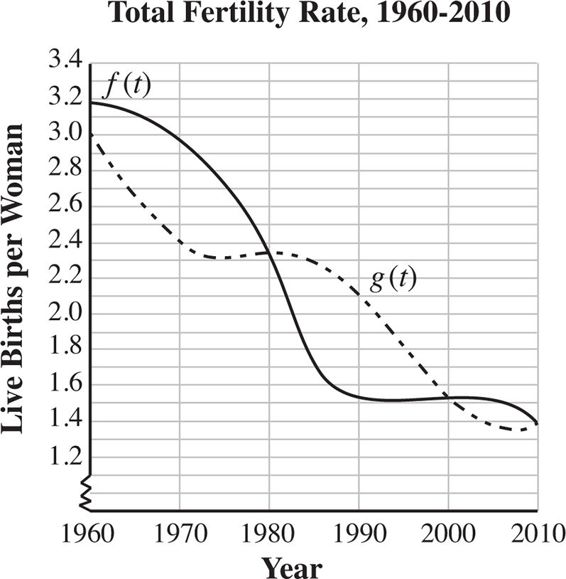# SAT Math Multiple Choice Question 306: Answer and Explanation

### Test Information

Question: 306

6.Source: Data from Eurostat.

One indicator of a declining economy is a continued decline in birth rates. In 2010, birth rates in Europe were at an all-time low, with the average number of children that a woman has in her lifetime at well below two. In the figure above, f(t) represents birth rates for Portugal between 1960 and 2010, and g(t) represents birth rates in Slovakia for the same time period. For which value(s) of t is f(t) > g(t)?

• A. 1960 < t < 1980 only
• B. 1980 < t < 2000 only
• C. 1960 < t < 1980 and 1990 < t < 2000
• D. 1960 < t < 1980 and 2000 < t < 2010